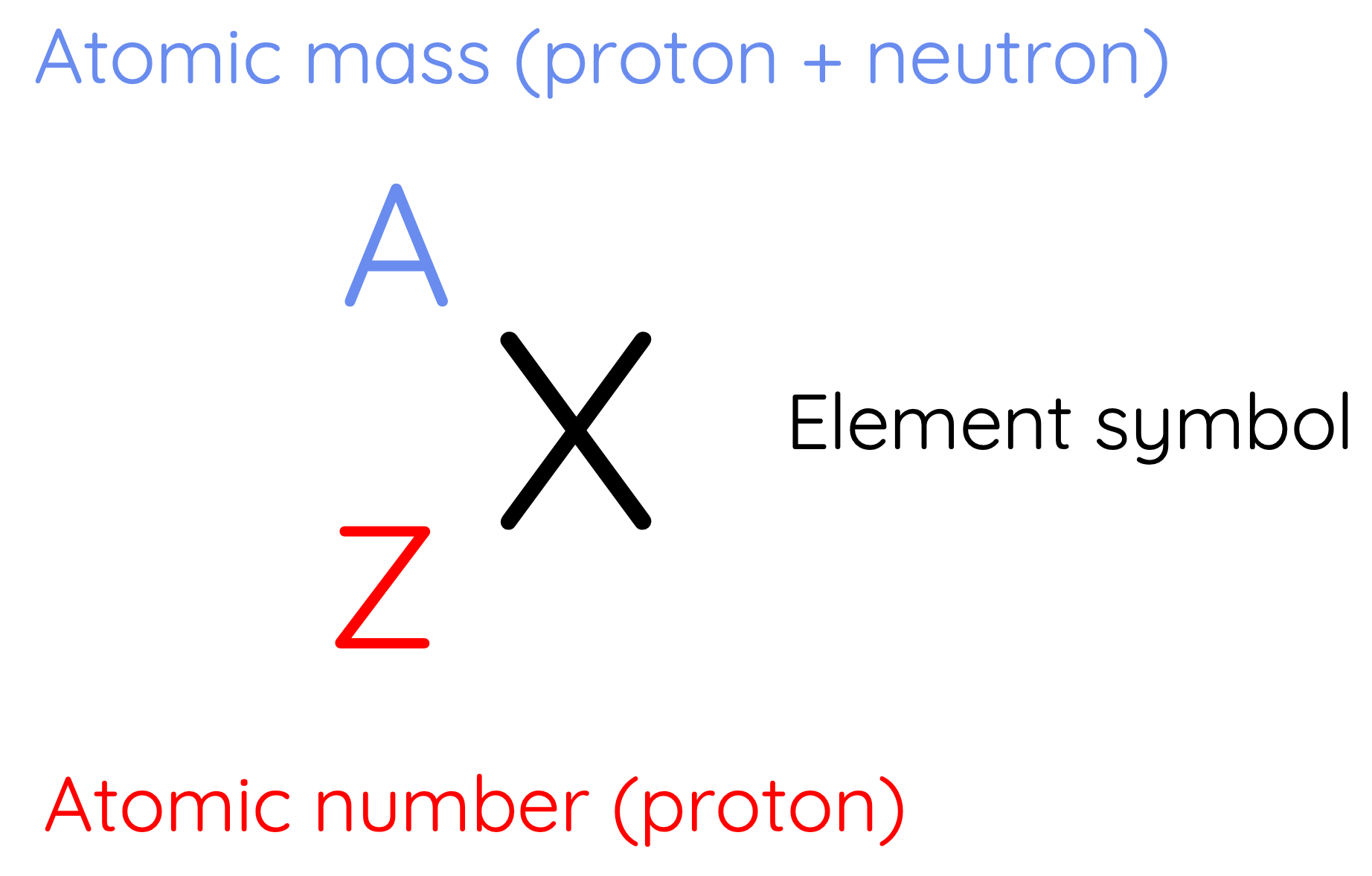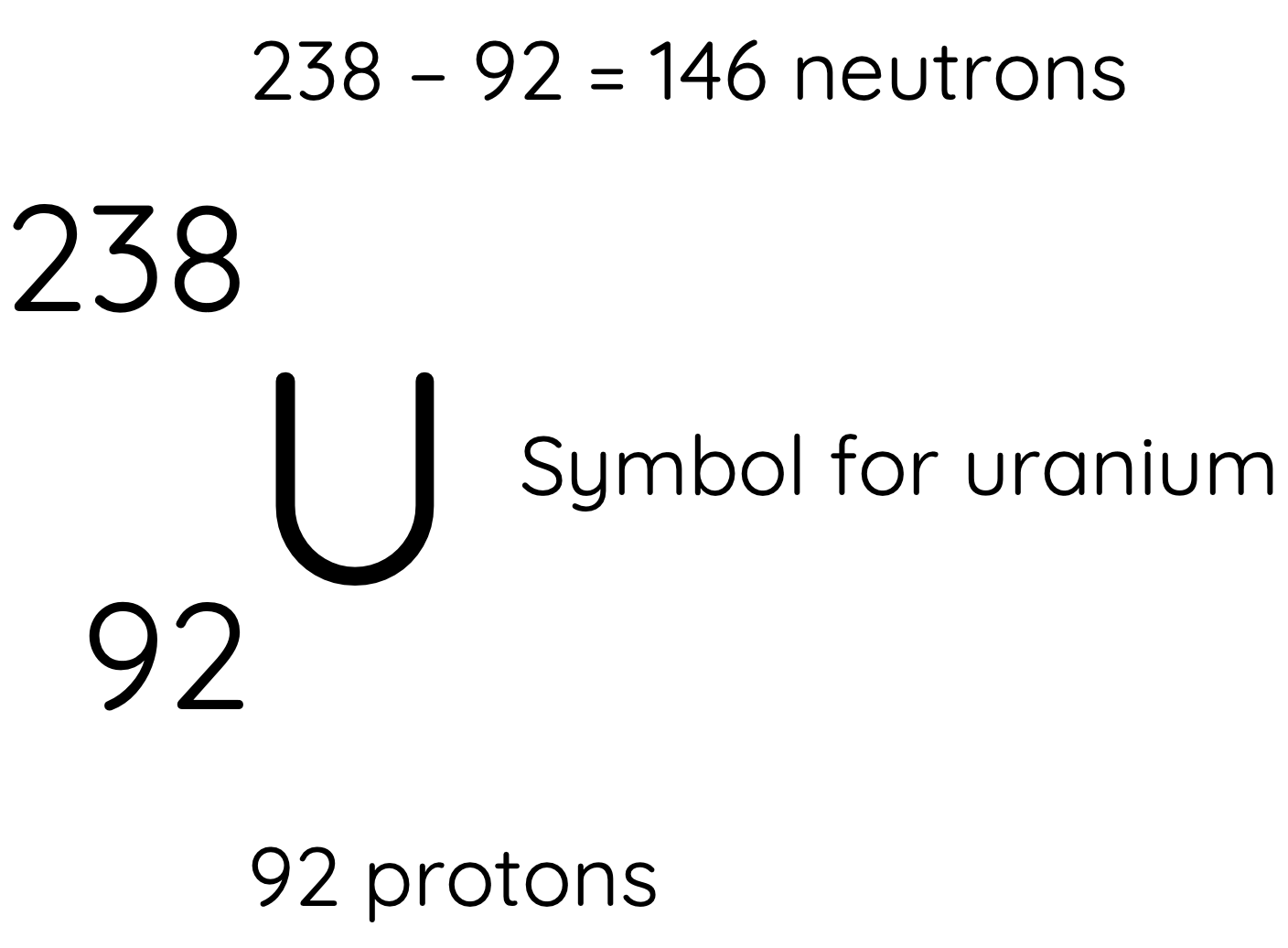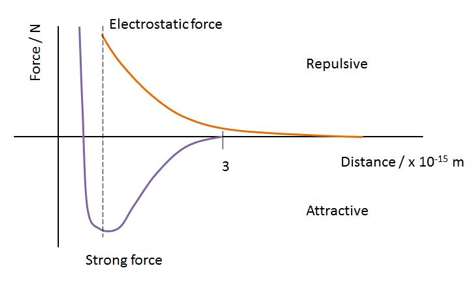# Strong Nuclear Force and Stability of the Nucleus

This topic is part of the HSC Physics course under the section Properties of the Nucleus.

### HSC Physics Syllabus

• analyse the spontaneous decay of unstable nuclei, and the properties of the alpha, beta and gamma radiation emitted (ACSPH028, ACSPH030)

### Nuclear Physics Nomenclature

The nucleus of an atom contains protons and neutrons, which are referred to as nucleons.

• Protons have positively charged while neutrons are uncharged
• Neutrons have slightly greater mass than protonsA nucleus has a unique nuclear nomenclature which consists of:

• atomic number is the number of protons in the nucleus
• atomic mass is the number of protons plus neutrons in the nucleus
• element symbol is found in the Periodic Table

The number of neutrons can be calculated by subtracting the atomic number from the atomic mass.

For example, uranium-238 (U-238):A nuclide is an atom or nucleus characterised by a specific number of protons and neutrons.

Isotopes are nuclides with the same number of protons but different number of neutrons. For example, U-238 and U-234 are isotopes of uranium because they both contain 92 protons but different number of neutrons.

### What are the forces inside the nucleus?

Electrostatic repulsion and gravitational attraction between masses in the nucleus are two forces that act between nucleons (protons and neutrons). The magnitude of electrostatic repulsion between protons is much greater than the gravitational attraction between the nucleons. In fact, the gravitational attraction between nucleons is so small it can be disregarded in most calculations involving the forces between nucleons.
From the calculations below, it can be seen that electrostatic repulsion vastly overcomes gravitational attraction between nucleons.### Strong Nuclear Force

Electrostatic repulsion between protons is overcome by strong nuclear force between nucleons. The stability of a nucleus is affected by the interaction between the strong nuclear force and electrostatic force.The magnitude and direction of strong nuclear force varies with the distance between two nucleons.

• It is attractive when nucleons are in normal distance from each other. This attractive force decreases with distance and becomes negligible beyond 2.5 fentometres.
• It is repulsive when the separation distance between two nucleons is lesser than 0.5-0.7 fentometres.
• It is greater in magnitude and opposite in direction to electrostatic force at typical nucleon separation. The greatest magnitude occurs when the separation distance is between 1-1.5 fentometres.Since strong force is only significant over very short distances, electrostatic force becomes the prominent force when nucleons are far apart. When the total strong nuclear force inside a nucleus is insufficient to balance the total electrostatic repulsion, radioactive decay occurs.

### What makes a nucleus unstable?

The stability of a nucleus is determined by its composition (number of protons and neutrons) because this determines the relative magnitude of strong force and electrostatic repulsion.

The number of protons in a nucleus determines the magnitude of electrostatic repulsion and strong nuclear force. The number of neutrons in a nucleus determines the magnitude of strong nuclear force.

Nuclei are naturally unstable because:

1. Large nuclei - As the number of nucleons in a nucleus increases, the distance between them inevitably becomes smaller. This causes the strong nuclear force to become more frequently repulsive. When the atomic number (Z) is greater than 83, the electrostatic repulsion between protons becomes so immense that almost all isotopes are unstable and hence can undergo radioactive decay.

2. Unstable neutron to proton ratio

As the number of protons in a nucleus increases, the total strong nuclear force and electrostatic repulsion both increase. However, the electrostatic repulsion generally increases more than the strong nuclear force as the latter is only present between protons separated by small distances. This difference in increase becomes more significant as the number of protons increases.

Therefore, additional neutrons are required to stabilise the nucleus as the number of proton increases. Adding neutrons to a nucleus increases the total magnitude of strong force between all nucleons.A is the atomic mass and Z is the atomic number (number of protons). Linear line shows a one-to-one ratio between neutrons and protons

For smaller elements, one neutron is needed for every proton to achieve nuclear stability, so a stable n/p ratio is 1:1

For larger elements, the stable n/p ratio becomes greater than 1 as increasing number of neutrons is required to balance the increasing magnitude of electrostatic repulsion between protons.

When the neutron to proton ratio is too high, the nucleus also becomes unstable because the average distance between nucleons will become too close, causing strong nuclear forces to convert from being attractive to repulsive.

Previous section: Schrodinger's Contribution to Model of the Atom